# Two uniform rods, ABC of weight 6 lb and DCE of weight 8 lb, are connected by a pin at C and by two cords BD and BE. The T-shaped assembly rotates in a vertical plane under the combined effect of gravity and of a couple M which is applied to rod ABC. Knowing that at the instant shown the tension in cord BE is 2 lb and the tension in cord BD is 0.5 lb, determine (a) the angular acceleration of the assembly, (b) the couple M. Fig. P16.88

Question-AnswerCategory: Engineering MechanicsTwo uniform rods, ABC of weight 6 lb and DCE of weight 8 lb, are connected by a pin at C and by two cords BD and BE. The T-shaped assembly rotates in a vertical plane under the combined effect of gravity and of a couple M which is applied to rod ABC. Knowing that at the instant shown the tension in cord BE is 2 lb and the tension in cord BD is 0.5 lb, determine (a) the angular acceleration of the assembly, (b) the couple M. Fig. P16.88

Two uniform rods, ABC of weight 6 lb and DCE of weight 8 lb, are connected by a pin at C and by two cords BD and BE. The T-shaped assembly rotates in a vertical plane under the combined effect of gravity and of a couple M which is applied to rod ABC. Knowing that at the instant shown the tension in cord BE is 2 lb and the tension in cord BD is 0.5 lb, determine (a) the angular acceleration of the assembly, (b) the couple M.
Fig. P16.88Step: 1

7949-16-88P AID: 1825 | 22/11/2013
Calculate the tangential acceleration of rod AC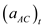.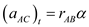Here, radius of rotation of rod AC is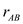, and angular acceleration of assembly is.
Substitute 9 in. for.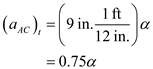Calculate the tangential acceleration of rod DE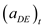.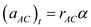Here, radius of rotation of rod DE is.
Substitute 18 in. for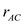.Calculate the moment of inertia of rod AC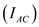.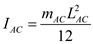Here, mass of rod AC is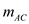, and length of rod AC is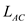.
Substitutefor, 6 lb for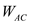, 32.2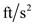for g, and 18 in. for.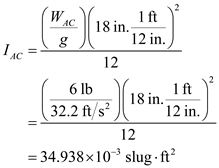Calculate the moment of inertia of rod DE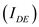.Here, mass of rod DE is, and length of rod DE is.
Substitute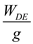for, 8 lb for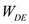, 32.2for g, and 24 in. for.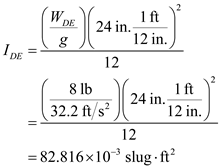Show the free body diagram of the given system as in Figure (1).Show the kinetic diagram of the given system as in Figure (2).Since the system of external forces is equivalent to system of effective forces, express the system of external moment as: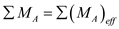…… (1)
Take counterclockwise moment as positive.
From Figure (1), calculate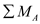.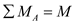Here, couple force applied to rod ABC is M.
From Figure (2), calculate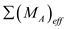.In Equation (1), substitute M for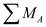, andfor.Substitutefor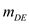, 8 lb for, 32.2for g,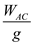for, 6 lb for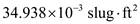for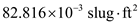for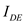for, andfor.…… (2)
Calculate the angle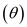between rod DE and cord BD.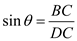Here, distance between point B and C is BC, and distance between point D and C is DC.
Substitute 9 in. for BC, and 12 in. for DC.Show the free body diagram of rod DE as in Figure (3).Show the kinetic diagram of rod DE as in Figure (4).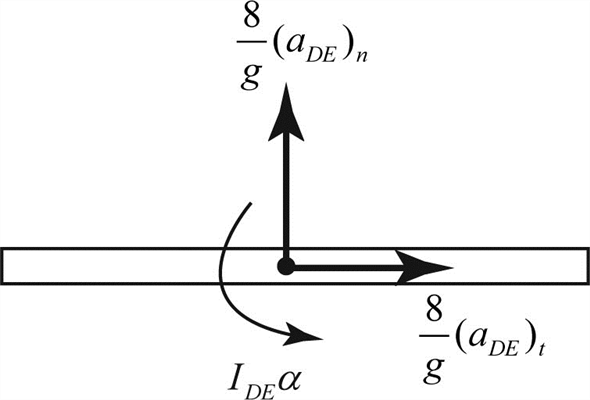a)
Since the system of external forces is equivalent to system of effective forces, express the system of external moments as:…… (3)
Take counterclockwise moment as positive.
From Figure (3), calculate.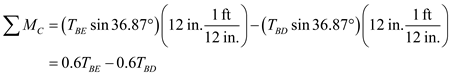Here, tension force in cord BE is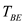, and tension force in cord BD is.
From Figure (4), calculate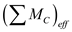.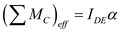Substitute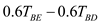for, andforin Equation (3).Substitute 2 lb for, 0.5 lb for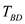, andfor.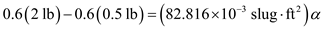Hence, the angular acceleration of assembly is.
b)
Substitutefor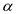in Equation (2).Hence, the magnitude of couple M is.